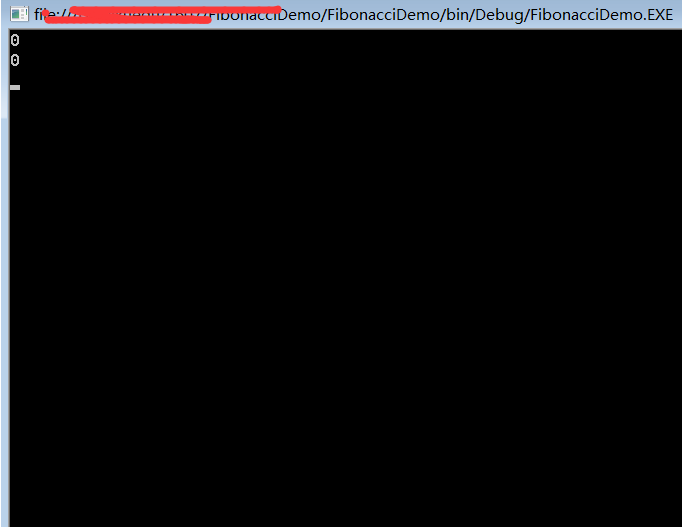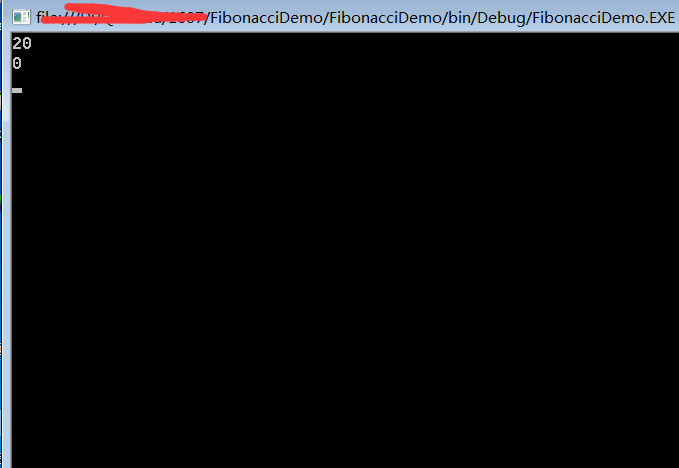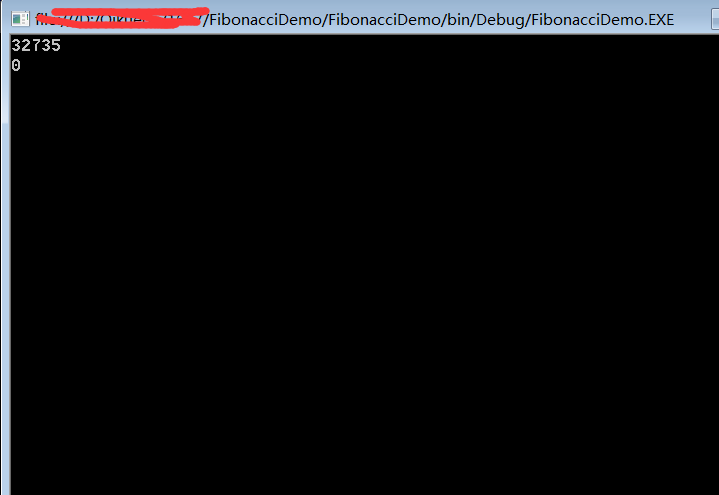# 由斐波那契数浅谈递归运行效率问题

using System;
using System.Collections.Generic;
using System.Linq;
using System.Text;

namespace FibonacciDemo
{
class Program
{
//递归
static long fibonacci_recursion(int n) {

if (n <= 2) {
return 1;
}
return fibonacci_recursion(n - 1) + fibonacci_recursion(n - 2);
}
//常规的迭代
static long fibonacci_iteration(int n) {
long result;
long previous_result;
long next_older_result;
result = previous_result = 1;

while (n > 2) {
n--;
next_older_result = previous_result;
previous_result = result;
result = previous_result + next_older_result;
}
return result;
}
static void Main(string[] args)
{
//在运行前获取一下系统时间
DateTime beforDT = System.DateTime.Now;
fibonacci_recursion(45);
//在运行前后获取一下系统时间
DateTime afterDT = System.DateTime.Now;
//计算前后的时间差
TimeSpan ts = afterDT.Subtract(beforDT);
Console.WriteLine(ts.TotalMilliseconds);

DateTime beforDT1 = System.DateTime.Now;
fibonacci_iteration(45);
DateTime afterDT1 = System.DateTime.Now;
TimeSpan ts1 = afterDT1.Subtract(beforDT1);
Console.WriteLine(ts1.TotalMilliseconds);

}
}
}fibonacci_recursion（4）
= fibonacci_recursion（3）+fibonacci_recursion（2）
=fibonacci_recursion（2）+fibonacci_recursion（1）+fibonacci_recursion（2）=3
fibonacci_recursion（8）
= fibonacci_recursion（7） + fibonacci_recursion（6）
=fibonacci_recursion（6）+fibonacci_recursion（5）+fibonacci_recursion（5） +fibonacci_recursion（4）
=fibonacci_recursion（5） +fibonacci_recursion（4） +fibonacci_recursion（3） +fibonacci_recursion（2） +fibonacci_recursion（3）+fibonacci_recursion（2）
+fibonacci_recursion（3） +fibonacci_recursion（2） =fibonacci_recursion（4） +fibonacci_recursion（3）+fibonacci_recursion（3） +fibonacci_recursion（2）+fibonacci_recursion（3） +fibonacci_recursion（2） +fibonacci_recursion（3）+fibonacci_recursion（2）
+fibonacci_recursion（3） +fibonacci_recursion（2） =21